### Home > CC1 > Chapter 7 > Lesson 7.1.3 > Problem7-34

7-34.
1. Compute each sum or difference. Homework Help ✎

1.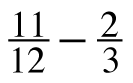2.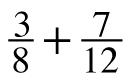3.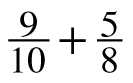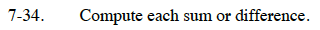Before adding or subtracting, change one or both fractions so that they have the same denominator.

Use a Giant One to convert to an equivalent fraction.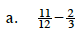Find the lowest common denominator.

(3)(4) = 12

Convert the fraction(s).

$\left ( \frac{2}{3} \right )\left ( \frac{4}{4} \right )=\frac{8}{12}$

Find the sum or difference.

$\frac{11}{12}-\frac{8}{12}=\frac{3}{12}$

Simplify, if necessary.

$\frac{3}{12}=\frac{1}{4}$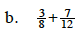$\frac{23}{24}$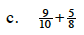The lowest common denominator is 40.